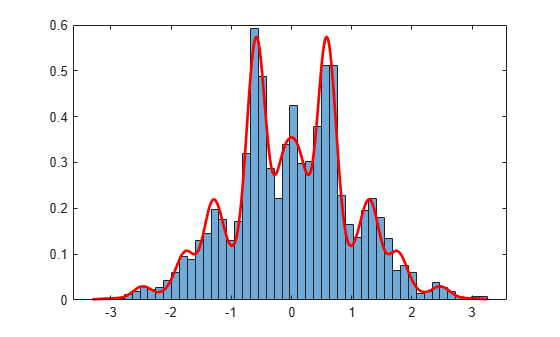# slicesample

## Syntax

```rnd = slicesample(initial,nsamples,'pdf',pdf) rnd = slicesample(initial,nsamples,'logpdf',logpdf) [rnd,neval] = slicesample(initial,...) [rnd,neval] = slicesample(initial,...,Name,Value) ```

## Description

`rnd = slicesample(initial,nsamples,'pdf',pdf)` generates `nsamples` random samples using the slice sampling method (see Algorithms). `pdf` gives the target probability density function (pdf). `initial` is a row vector or scalar containing the initial value of the random sample sequences.

`rnd = slicesample(initial,nsamples,'logpdf',logpdf)` generates samples using the logarithm of the pdf.

```[rnd,neval] = slicesample(initial,...)``` returns the average number of function evaluations that occurred in the slice sampling.

```[rnd,neval] = slicesample(initial,...,Name,Value)``` generates random samples with additional options specified by one or more `Name,Value` pair arguments.

## Input Arguments

 `initial` Initial point, a scalar or row vector. Set `initial` so `pdf(initial)` is a strictly positive scalar. `length(initial)` is the number of dimensions of each sample.
 `nsamples` Positive integer, the number of samples that `slicesample` generates.
 `pdf` Handle to a function that generates the probability density function, specified with `@`. `pdf` can be unnormalized, meaning it need not integrate to `1`.
 `logpdf` Handle to a function that generates the logarithm of the probability density function, specified with `@`. `logpdf` can be the logarithm of an unnormalized pdf.

### Name-Value Arguments

Specify optional pairs of arguments as `Name1=Value1,...,NameN=ValueN`, where `Name` is the argument name and `Value` is the corresponding value. Name-value arguments must appear after other arguments, but the order of the pairs does not matter.

Before R2021a, use commas to separate each name and value, and enclose `Name` in quotes.

 `burnin` Nonnegative integer, the number of samples to generate and discard before generating the samples to return. The slice sampling algorithm is a Markov chain whose stationary distribution is proportional to that of the `pdf` argument. Set `burnin` to a high enough value that you believe the Markov chain approximately reaches stationarity after `burnin` samples. Default: `0`
 `thin` Positive integer, where `slicesample` discards every `thin - 1` samples and returns the next. The slice sampling algorithm is a Markov chain, so the samples are serially correlated. To reduce the serial correlation, choose a larger value of `thin`. Default: `1` `width` Width of the interval around the current sample, a scalar or vector of positive values. `slicesample` begins with this interval and searches for an appropriate region containing the points of `pdf` that evaluate to a large enough value. If `width` is a scalar and the samples have multiple dimensions, `slicesample` uses `width` for each dimension.If `width` is a vector, it should have the same length as `initial`. Default: `10`

## Output Arguments

 `rnd` `nsamples`-by-`length(initial)` matrix, where each row is one sample.
 `neval` Scalar, the mean number of function evaluations per sample. `neval` includes the `burnin` and `thin` evaluations, not just the evaluations of samples returned in `rnd`. Therefore the total number of function evaluations is `neval*(nsamples*thin + burnin)`.

## Examples

collapse all

This example shows how to generate random samples from a multimodal density using `slicesample`.

Define a function proportional to a multimodal density.

```rng default % For reproducibility f = @(x) exp(-x.^2/2).*(1 + (sin(3*x)).^2).*... (1 + (cos(5*x).^2)); area = integral(f,-5,5);```

Generate 2000 samples from the density, using a burn-in period of 1000, and keeping one in five samples.

```N = 2000; x = slicesample(1,N,'pdf',f,'thin',5,'burnin',1000);```

Plot a histogram of the sample.

```[binheight,bincenter] = hist(x,50); h = bar(bincenter,binheight,'hist'); h.FaceColor = [.8 .8 1];```Scale the density to have the same area as the histogram, and superimpose it on the histogram.

```hold on h = gca; xd = h.XLim; xgrid = linspace(xd(1),xd(2),1000); binwidth = (bincenter(2)-bincenter(1)); y = (N*binwidth/area) * f(xgrid); plot(xgrid,y,'r','LineWidth',2) hold off```The samples seem to fit the theoretical distribution well, so the `burnin` value seems adequate.

## Tips

• There are no definitive suggestions for choosing appropriate values for `burnin`, `thin`, or `width`. Choose starting values of `burnin` and `thin`, and increase them, if necessary, to give the requisite independence and marginal distributions. See Neal  for details of the effect of adjusting `width`.

## Algorithms

At each point in the sequence of random samples, `slicesample` selects the next point by “slicing” the density to form a neighborhood around the previous point where the density is above some value. Consequently, the sample points are not independent. Nearby points in the sequence tend to be closer together than they would be from a sample of independent values. For many purposes, the entire set of points can be used as a sample from the target distribution. However, when this type of serial correlation is a problem, the `burnin` and `thin` parameters can help reduce that correlation.

`slicesample` uses the slice sampling algorithm of Neal . For numerical stability, it converts a `pdf` function into a `logpdf` function. The algorithm to resize the support region for each level, called “stepping-out” and “stepping-in,” was suggested by Neal.

## References

 Neal, Radford M. "Slice Sampling." Ann. Stat. Vol. 31, No. 3, pp. 705–767, 2003. Available at Project Euclid.

## Version History

Introduced in R2006a